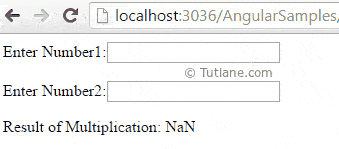# AngularJS ng-model Directive with Example

Here we will learn ng-model directive in angularjs with example, uses of ng-model directive and how to use ng-model directive in angularjs with example.

## AngularJS ng-model Directive

In angularjs `ng-model` directive is used to get value of input controls like textbox, label, etc and bind that value to application data. Generally, the syntax of `ng-model` directive in angularjs like as shown below.

## Syntax of ng-model Directive in AngularJS

Following is the syntax of using `ng-model` directive in angularjs applications.

<div ng-app="">

<input type="text" id="txtname" ng-model="name" />

</div>

If you observe the above syntax, we declared `ng-model="name"` for textbox controller because of that whenever the user type text in the textbox control we can get that value use it whever it required. We will see complete example of ng-model directive in angularjs

## Example of AngularJS ng-model Directive

Following is the example of using `ng-model` directive in angularjs applications.

<html xmlns="http://www.w3.org/1999/xhtml">

<title>

AngularJs ng-model Directive example

</title>

<body>

<div ng-app="">

Enter Number1:<input type="text" ng-model="number1" /><br /><br />

Enter Number2:<input type="text" ng-model="number2" />

<p>Result of Multiplication: {{number1 * number2}}</p>

</div>

</body>

</html>

If you observe the above code, we are getting two textbox control values by using `ng-model` property and performing the multiplication operation on it.

## Output of ng-model

When we execute the above `ng-model` directive example, we will get the result as shown below.This is how we can `ng-model` directive in angularjs application to get input control values and we can use for required operations.